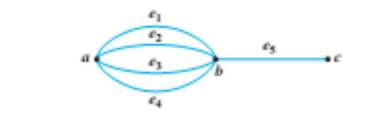Chapter 10.1, Problem 5ES### Discrete Mathematics With Applicat...

5th Edition
EPP + 1 other
ISBN: 9781337694193

#### Solutions

Chapter
Section### Discrete Mathematics With Applicat...

5th Edition
EPP + 1 other
ISBN: 9781337694193
Textbook Problem
6 views

# Consider the following graph. How many paths are there from a to c? How many trails are there from a to c? How many walks are there from a to c?To determine

(a)

How many paths are there from a to c?

Explanation

Given information:

Consider the following graph.

Calculation:

A path is a walk from a to c with no repeating edges. a and c can follow 4 different paths:

ae1be5</

To determine

(b)

How many trails are there from a to c?

To determine

(c)

How many walks are there from a to c?

### Still sussing out bartleby?

Check out a sample textbook solution.

See a sample solution

#### The Solution to Your Study Problems

Bartleby provides explanations to thousands of textbook problems written by our experts, many with advanced degrees!

Get Started